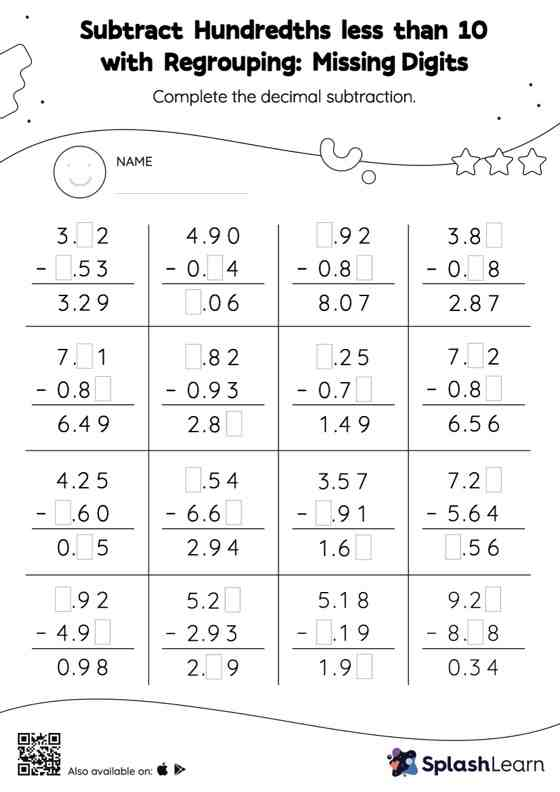# Subtract Hundredths less than 10 with Regrouping: Missing Digits Worksheet

Home > Subtract Hundredths less than 10 with Regrouping: Missing DigitsThis worksheet challenges young mathematicians to hone their math skills by solving a set of problems on subtracting hundredths less than 10 with regrouping. In each problem, the numbers are laid out in the vertical format. In this format, pair of digits in each successive place are tackled one by one. This helps students follow a structured approach.